×
Get Full Access to Introductory Chemistry - 5 Edition - Chapter 10 - Problem 76p
Get Full Access to Introductory Chemistry - 5 Edition - Chapter 10 - Problem 76p

×

Determine the molecular geometry of each polyatomic ion.(a) ClO4?(b) BrO2?(c) NO2?(d)ISBN: 9780321910295 34

Solution for problem 76P Chapter 10

Introductory Chemistry | 5th Edition

• Textbook Solutions
• 2901 Step-by-step solutions solved by professors and subject experts
• Get 24/7 help from StudySoup virtual teaching assistantsIntroductory Chemistry | 5th Edition

4 5 1 396 Reviews
17
0
Problem 76P

PROBLEM 76P

Determine the molecular geometry of each polyatomic ion.

(a) ClO4−

(b) BrO2−

(c) NO2−

(d) SO42−

Step-by-Step Solution:

Problem 76P

Determine the molecular geometry of each polyatomic ion.

(a)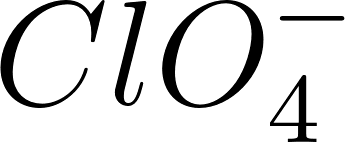(b)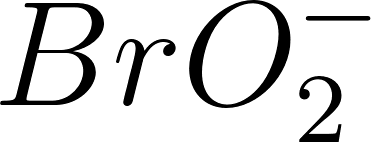(c)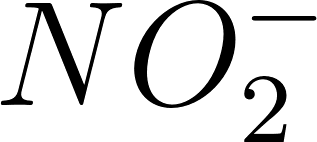(d)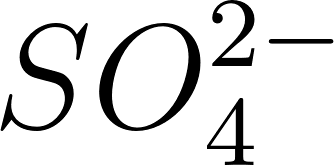Step by Step Solution

Step 1 of 4

Molecular geometry used to describe the shape of a molecule.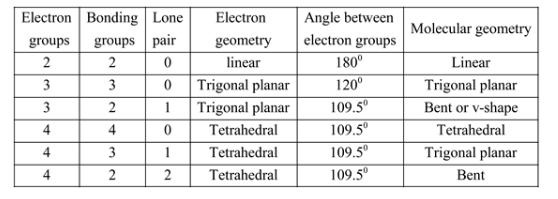The given polyatomic ion -.

Name of the polyatomic ion - Chlorate ion.

The structure ofis as follows.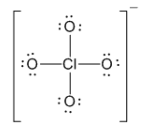In the given ion, the central atom - chlorine.

And it is attached to the four groups which all are bonding groups.

Hence, it has 4 - electron groups and 4- bonding groups.

From the above table, if the molecule has 4 electrons groups and 4 bonding, the shape is tetrahedral in both electron and molecular geometry.

The molecular geometry ofis shown below.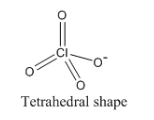Therefore, the molecular geometry of a chlorate ion is tetrahedral.

Step 2 of 4

Step 3 of 4

ISBN: 9780321910295

Introductory Chemistry was written by and is associated to the ISBN: 9780321910295. This textbook survival guide was created for the textbook: Introductory Chemistry, edition: 5. Since the solution to 76P from 10 chapter was answered, more than 427 students have viewed the full step-by-step answer. The answer to “Determine the molecular geometry of each polyatomic ion.(a) ClO4?(b) BrO2?(c) NO2?(d) SO42?” is broken down into a number of easy to follow steps, and 12 words. This full solution covers the following key subjects: bro, clo, determine, geometry, ion. This expansive textbook survival guide covers 19 chapters, and 2046 solutions. The full step-by-step solution to problem: 76P from chapter: 10 was answered by , our top Chemistry solution expert on 05/06/17, 06:45PM.

Unlock Textbook Solution